# McGraw Hill Math Grade 4 Chapter 2 Lesson 4 Answer Key More Practice with Adding Whole Numbers

Practice the questions of McGraw Hill Math Grade 4 Answer Key PDF Chapter 2 Lesson 4 More Practice with Adding Whole Numbers to secure good marks & knowledge in the exams.

## McGraw-Hill Math Grade 4 Answer Key Chapter 2 Lesson 4 More Practice with Adding Whole Numbers

Question 1.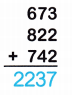Explanation:
Sum of 673, 822 and 742:
673+ 822 +742
= 1,495 + 742
= 2,237.

Question 2.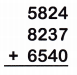Explanation:
Sum of 5824, 8237 and 6540:
5,824 + 8,237 + 6,540
= 14,061 + 6540
= 20,601.

Question 3.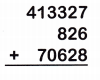Explanation:
Sum of 413327, 826 and 70628:
413,327 + 826 + 70,628
= 414,513 + 70,628
= 484,781.

Question 4.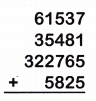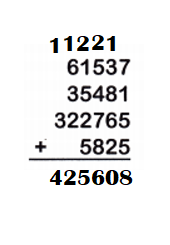Explanation:
Sum of 61,537, 35,481, 322,765 and 5,825:
61,537 + 35,481 + 322,765 + 5,825
= 97,018 + 322,765 + 5,825
= 419783 + 5,825
= 425,608.

Question 5.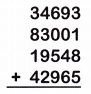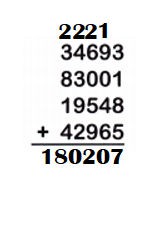Explanation:
Sum of 34,693, 83,001, 19,548 and 42,965:
34,693 + 83,001 + 19,548 + 42,965
= 117,694 + 19,548 + 42,965
= 137,242 + 42,965
= 180,207.

Question 6.Explanation:
Sum of 512,868, 5,906, 124,850 and 257,623:
512,868 + 5,906 + 124,850 + 257,623
= 518,774 + 124,850 + 257,623
= 643,624 + 257,623
= 901,247.

Question 7.
Clearwater, Florida, had a population of 107,685 in 2010. Coral Springs had a population of 121,096. Orlando had a population of 238,300. What was the total population of the three communities?